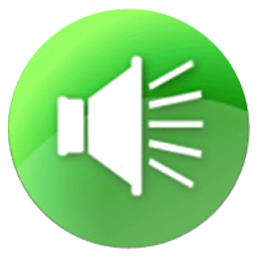These “OUR” spellings say the “ER” sound:

 Rotated R
Rotated RC o u r a g e

Rotated RA d j o u r n

Rotated RG l a m o u r

Rotated RN o u r i s h

Rotated RJ o u r n e y

But these “OUR” spellings say the “HOUR” sound:

 Combined
CombinedO u r s

CombinedS o u r b a l l

CombinedH o u r l y

CombinedF l o u r

CombinedS c o u r

Good grief, these “OUR” spellings say the “OAR” sound:

 Blended
BlendedF o u r

BlendedP o u r e d

BlendedC o u r t h o u s e

BlendedM o u r n f u l

BlendedG o u r d s

Oh my, and look at OTHER ways we spell the “OAR” sound, in addition to OUR — OR, ORE, OAR, EOR, OOR:
(Even OHR: Bohr, bohrium)

 Blended
BlendedM o r e

BlendedS t o r e

BlendedR o a r

BlendedS o a r

BlendedG e o r g e

BlendedG e o r g i a

BlendedD o o r

BlendedF l o o r

BlendedN o r t h

BlendedF o r k

BlendedF o r

BlendedO r

You’ve gotta be kidding. Now you tell me that “OR” can sound different than “OAR?” It can make the “ER” sound?

 Rotated R
Rotated RC o l o r

Rotated RD o c t o r

Rotated RA u t h o r

Rotated RR a z o r

Rotated RH o r r o r

Rotated RM o t o r

Rotated RA l l i g a t o r

Rotated RV e l o c i r a p t o r